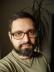## Kth Permutation Sequence

Write a script to accept two integers n (>=1) and k (>=1). It should print the k-th permutation of n integers.

For example, n=3 and k=4, the possible permutation sequences are listed below:

```123
132
213
231
312
321```

The script should print the 4th permutation sequence 231.

The straightforward way is to generate all the permutations in the correct order and then output the kth one. To generate them, we can use recursion: To get all the permutations of n elements, start with each element and extend it with all the permutations of the remaining elements.

``````#!/usr/bin/perl
use warnings;
use strict;
use feature qw{ say };

sub perm {
my (\$src) = @_;
return [\$src] if 1 == @\$src;

my @perms;
for my \$s (@\$src) {
my \$subperms = perm([grep \$s != \$_, @\$src]);
push @perms, map [\$s, @\$_], @\$subperms;
}
return \@perms
}

sub kth_perm {
my (\$n, \$k) = @_;
return @{ perm([1 .. \$n])->[\$k - 1] }
}

my (\$n, \$k) = @ARGV;
say kth_perm(\$n, \$k);``````

The problem of this solution is performance. It takes it more than 2 seconds to output the 5678th permutation of 1 .. 9. If we replace the `!=` with `ne` so we can process permutations of letters, too, it takes twice as more.

There is a way how to construct the kth permutation directly without enumerating the previous ones. Let’s have a closer look: The first character divides all the permutations into n groups.

```1234   2134   3124   4123
1243   2143   3142   4132
1324   2314   3214   4213
1342   2341   3241   4231
1423   2413   3412   4312
1432   2431   3421   4321```

Size of each group is the number of permutations of n - 1 elements (i.e. the characters following the first one). And the same applies to subgroups of each group, e.g.

```1234   1324   1423
1243   1342   1432```

Size of each group is the number of permutations of the remaining elements.

The number of all the permutations of x elements is x! or x factorial, i.e. `product(1 .. \$x)`. If the k is given, e.g. 15, we can divide it by the size of a subgroup to know what group we can find the result in (`int(15 / 6) == 3`). To repeat the step recursively, we need to know what k will be used in the subgroup. If we try searching several times, we’ll realise it’s the remainder of the division, i.e. `15 % 6 == 3` (in the code bellow, you can notice I simplified the border conditions).

``````#!/usr/bin/perl
use warnings;
use strict;
use feature qw{ say };

use List::Util qw{ product };

sub perm_recurse {
my (\$k, @n) = @_;
return "" unless @n;

my \$factorial = product(1 .. @n);
my \$step = \$factorial / @n;

my \$select = int(\$k / \$step);
--\$select unless \$k % \$step;

return \$n[\$select]
. perm_recurse((\$k % \$step) || \$step,
@n[ grep \$_ != \$select, 0 .. \$#n ])
}

sub kth_perm { perm_recurse(\$_, 1 .. \$_) }

my (\$n, \$k) = @ARGV;
say kth_perm(\$n, \$k);``````

This code finds the 5678th permutations of 9 elements in less than 0.01 seconds. Even calculating `perm_recurse(10, 'a' .. 'z')` takes less than a 0.1 seconds, not talking about the memory consumption of the naive approach.

## Collatz Conjecture

It is thought that the following sequence will always reach 1:
```\$n = \$n / 2 when \$n is even
\$n = 3*\$n + 1 when \$n is odd```

For example, if we start at 23, we get the following sequence:

`23 → 70 → 35 → 106 → 53 → 160 → 80 → 40 → 20 → 10 → 5 → 16 → 8 → 4 → 2 → 1`

Write a function that finds the Collatz sequence for any positive integer. Notice how the sequence itself may go far above the original starting number.

### Extra Credit

Have your script calculate the sequence length for all starting numbers up to 1000000 (1e6), and output the starting number and sequence length for the longest 20 sequences.

I started with the naive implementation again. This time, there’s not trick, including the extra credit: just enumerate all the sequences, remember them in an array, sort them by size and output the top 20.

``````#!/usr/bin/perl
use warnings;
use strict;
use feature qw{ say };

sub collatz {
my (\$start) = @_;
my @seq = \$start;
push @seq, (\$seq[-1] / 2, 3 * \$seq[-1] + 1)[\$seq[-1] % 2]
while \$seq[-1] != 1;
return @seq
}

my @sizes;
push @sizes, [\$_, scalar collatz(\$_)] for 1 .. 1e6;
say "@\$_" for reverse +(sort { \$b-> <=> \$a-> } @sizes)[0 .. 19];``````

I was worried about the performance again. The program took 38 seconds to compute the extra credit task which didn’t sound fast. I tried to only keep a heap of the 20 longest sequences instead of storing all of them, but it was in fact even slower. I also compared my solution to Laurent’s one, realising dynamic programming would shave off about 6 seconds (about 15%). I wasn’t sure such a small gain was worth the effort, so I stopped there.I blog about Perl.